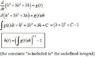# Nonlinear 1o Order D.E.

pvgomes07
Hello!

I am taking a self study diff e course, and I have run into a problem with no one to ask for help.
Here is the problem:

d/dt [ h^3(t) + 3h(t)^2 + 3h(t) ] = q(t)

h(t) is output.
q(t) is input.

is this Nonlinear First Order Differential Equation.
But I could not expand to Taylor Series for linearization... :/

I'm trying to find the transfer function.

Thanks!

Homework Helper
It should be basic Calculus that
$$\frac{d}{dx}(h^3+ 3h^2+ 3h)= q(t)$$
$$3h^2\frac{dh}{dt}+ 6h\frac{dh}{dt}+ 3\frac{dh}{dt}= (3h^2+ 6h+ 3)\frac{dh}{dt}= q(t)$$
Now what is the linearization of that?

Mentor
Hello!

I am taking a self study diff e course, and I have run into a problem with no one to ask for help.
Here is the problem:

d/dt [ h^3(t) + 3h(t)^2 + 3h(t) ] = q(t)

h(t) is output.
q(t) is input.

is this Nonlinear First Order Differential Equation.
But I could not expand to Taylor Series for linearization... :/

I'm trying to find the transfer function.

Thanks!

Maybe this will help:

h3 + 3 h2 + 3h = (h +1)3 -1

JJacquelin
Hi !
Why not imtegrate it first ?

#### Attachments

•Simple ODE.JPG
12.6 KB · Views: 391
pvgomes07
Hi !
Why not imtegrate it first ?

Yeah! Thank you very much!
:)